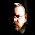Sunday, July 06, 2008

Drools and Machine Learning

I'm Gizil. I am doing my master thesis in Drools project. I'm working on decision trees. I have made an ID3, C4.5 implementation with rule generation. I'm investigating bagging and boosting algorithm in order to produce better rules.

I am using Annotations on object fields to be able to process extra information on the attributes of the objects. I'm generating the rules out of the trees by parsing the trees using depth first search and compiling with PackageBuilder before adding to the RuleBase. In the future I consider using MVEL for templating to generate the rules from the trees.

Target Attribute or Class Label

Since I implement a Supervised learning algorithm the class labels of the target class has to be given by the user. There are two ways to input the labels. The first and easy way is specifying one of the fields related to the target class as the label by the Annotation on that field. The second way is writing a getter function on the object class and specifiying by its Annotation.

Attribute Domains

The most common domain types are categorical and quantitative. Moreover, the decision trees need to deal with complex domains which are not simple primitive object types.
1. Categorical (discrete) domain is commonly constructed by a set of String values. There has to be a finite number of discrete values. The attributes are assumed to be categorical by default. Only if there is an annotation saying the opposite then the domain is treated as quantitative. The target attribute has to be categorical since it is not a regression tree implementation.

2. Quantitative (continuous) domain: Commonly, subset of real numbers, where there is a measurable difference between the possible values. Integers are usually treated as continuous in practical problems. This type of domain has to be discretized by defining a various number of thresholds (intervals) for each possible class. My implementation can discretize numerical attributes which are a set of real numbers and have quantitative domain.
3. For example: age < 15 as child
15 <= age < 20 as teenage
20 <= age as adult
Literal attributes which are set of Strings and have a continuous domain can also be discretized by defining a various number of sets for each possible class.
For example: letter element of {a, e, i, o, u} as vowel
letter not element of {a, e, i, o, u} as consonant

4. Complex domain implements a domain of an attribute that belongs to another object class. This type of domain needs more care because there are many possibilities such as Collections or references to the object class, itself.
Quinlan's C4.5 Algorithm

Comparing to the ID3 the C4.5 learning algorithm can tackle with harder domains that contain many number of possible values.
C4.5 deals with the numeric (integer or real) and continuous attributes using a discretization technic based on entropy.

Continuous Attribute Discretization

There are mainly two approaches of discretizing the continuous attributes. One approach is using a global discretization algorithm, which results in a smaller decision tree. A global discretization algorithm would ignore the relation of the continuous attribute with the other attributes. The other approach is at any node of tree dicretizing the continuous attribute on the current set of instances that means applying the global discretization algorithm during the training of the decision tree.

I implemented the Fayyad and Irani's the Minimum descriptive length method to discretize the numerical domains which is also used by the WEKA project. Fayyad and Irani used information gain approach while evaluating the effectiveness of the discretization. I also tried the Quinlan's gain ratio approach as an MDL method which is presented as a bit more fair evaluation of the domains due the normalization of the information gain by the information of the current data set based on the domain attribute. Moreover, there are some other approaches such as gini coefficient, or chi-squared test that need to be tested.

For example:  Using the 15 Golf instances with 4 attributes (1 boolean, 1 literal and 2 numerical = 2 Categorical and 2 Quantitative) and Boolean target attribute I get a rule saying that the decision should be to play golf outside if the outlook attribute of the Golf object is "overcast". This rule's rank is 0.2858 which means that the rule is classifiying 28.58 % of the given Golf objects.

rule "#0 decision= Play  classifying 4.0 num of facts with rank:0.2858"
when
\$golf_0 : Golf(outlook == "overcast", \$target_label : decision )
then
System.out.println("[decision] Expected value (" + \$target_label + "), Classified as (Play )");
end1.If you haven't come across this blog on machine learning, I would recommend reading it.

http://hunch.net/

the focus is on machine learning and has some good information.

2.Interesting post.

-Will Dwinnell
Data Mining in MATLAB

3.This comment has been removed by the author.

4.These are as yet headline news. Business pioneer or basic representative? machine learning certification

5.